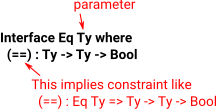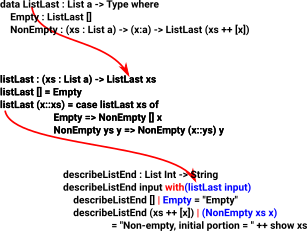# Idris Language

## User Defined Types

A user defined type is constructed using the 'data' keyword.

The simplest form just lists the constructors.

 `data PrimaryColour = Red | Green | Blue`

These type constructors can have parameters:

 `data Point2D = Pt Double Double`

We can also use 'where' syntax:

 ```data Point2D : Type where Pt : Double -> Double -> Point2D```

If we want to define Point types in 3 or more dimensions then, we could either repeat the definition

 ```data Point : Nat ->Type where Nil : Point Z (::) : Double -> Point k -> Point (S k)```

So, in the first case the type would just be referred to by name 'Point2D' in the second case the type name must always include a number 'Point 2'.

'Point', on its own is not a type, it might be known as a 'type constructor'.

### Generic Data Types

A type that's parameterised over some other type (type depends on type).

Type is represented by variable name starting with lower case.

 ```data Maybe valtype = Nothing | Just valtype```

## Idris Polymorphism

Implemented by interface.

This is a type spec which must be implemented by implementations of the interface.

 Example EqA specific implementation: ```Eq Colour where Red Red = True Blue Blue = True Green Green = True _ _ = False ```

Use

x==Red

(pattern match)

### Parametric Polymorphism

Example: Functor